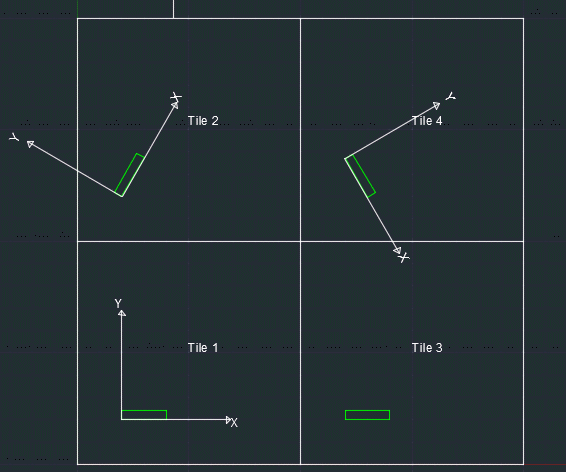## Area Fill - Calculating Polygon Perimeter

Areafill is used to characterize CAD layouts with respect to density and "directionality".
The layout or a user specified window is broken up into tiles. Density is a measure of metal coverage on a per tile basis.
In addition, the "dominant" direction is computed for each tile. The dominant direction is then used to provide the
sum of the length of the edges along and perpendicular to the dominant direction, i.e. the x-perimeter and y-perimeter values respectively.

Let's look at the following GDSII example file. In this file, there are 4 polygons that are 5x1 microns -
two of them are at 0 angle, one at 60 degree, and another at -60 degrees relative to the X axis.
The polygons are 25 microns apart along X and Y, and we will use tile size of 25x25 when using areafill.
Therefore, the output text file from areafill will include the perimeter and the angle of only one polygon per tile.### Example Command Line

We will run the tool from the command line and create two output text files.
One will be the standard output with the polygon area in each tile.
The second example will also include the perimeter calculation.

#### Example 1

In the first example, the output text file includes the total boundary area and the metal density of each tile.
```/home/cad/areafill/bin/areafill64 test.gds test_std.txt test +0 -tile:25,25 -falseperim
```
Here is how the output text file looks like:
```================
Tile Information
================

Number of tiles:   4
Number of rows:    2
Number of columns: 2
Tile width:        25 um
Tile height:       25 um
Tile area:         625 um^2
Window area:       2.5e+03 um^2

Job:   1
Layer: 0

Tile #    Row Column             Tile center (x,y)       Filled area (um^2)      % Relative

1      1      1           (7.067000,7.415000)                    5.000          0.800
2      2      1          (7.067000,32.415000)                    5.000          0.800
3      1      2          (32.067000,7.415000)                    5.000          0.800
4      2      2         (32.067000,32.415000)                    5.000          0.800
```

#### Example 2

In this example, the output file also includes the perimeter of each tile and the dominant angle of the polygons.
```/home/cad/areafill/bin/areafill64 test.gds test_per.txt test +0 -tile:25,25 -perimeter -falseperim
```
Here is how the output text file looks like:
```===================================
Dominant Direction Tile Information
===================================

Number of tiles:   4
Number of rows:    2
Number of columns: 2
Tile width:        25 um
Tile height:       25 um
Tile area:         625 um^2
Window area:       2.5e+03 um^2
Max perimeter Xdir:   10 um
Max perimeter Ydir:   2 um
Normalized perimeter: 10 um

Job:   1
Layer: 0

Tile #    Row Column             Tile center (x,y)       Filled area (um^2)      % Relative          Xperimeter (um)     % XRelative          Yperimeter (um)     % YRelative      Direction

1      1      1           (7.067000,7.415000)                    5.000          0.800                   10.000        100.000                    2.000         20.000            0.0
2      2      1          (7.067000,32.415000)                    5.000          0.800                   10.000         99.998                    2.000         20.001           60.0
3      1      2          (32.067000,7.415000)                    5.000          0.800                   10.000        100.000                    2.000         20.000            0.0
4      2      2         (32.067000,32.415000)                    5.000          0.800                   10.000         99.998                    2.000         20.001          -60.0
```

As you can see, the perimeter information along X and Y is the same in the 4 tiles.
That is because the the X/Y axis is rotating with the tile Direction value.
In this example, tiles 1 and 3 have an X axis that goes horizontal from left to right.
Tiles 2 and 4 have an X axis that is aligned with the tile direction, namely at 60 and -60 degrees (see image below).Download   |   Installation   |   Documentation   |   Command Line Syntax   |   Revision History